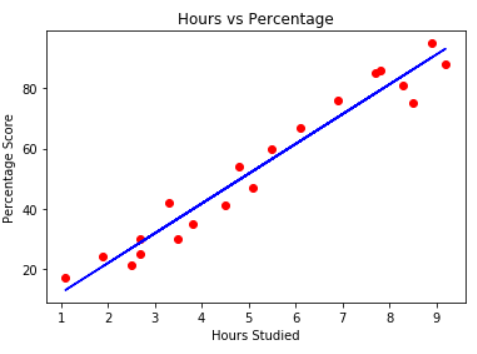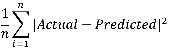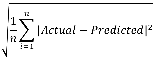Linear Regression in Python with Scikit-Learn - Stack Abuse

# Linear Regression in Python with Scikit-LearnThere are two types of supervised machine learning algorithms: Regression and classification. The former predicts continuous value outputs while the latter predicts discrete outputs. For instance, predicting the price of a house in dollars is a regression problem whereas predicting whether a tumor is malignant or benign is a classification problem.

In this article we will briefly study what linear regression is and how it can be implemented using the Python Scikit-Learn library, which is one of the most popular machine learning libraries for Python.

### Linear Regression Theory

The term "linearity" in algebra refers to a linear relationship between two or more variables. If we draw this relationship in a two dimensional space (between two variables, in this case), we get a straight line.

Let's consider a scenario where we want to determine the linear relationship between the numbers of hours a student studies and the percentage of marks that student scores in an exam. We want to find out that given the number of hours a student prepares for a test, about how high of a score can the student achieve? If we plot the independent variable (hours) on the x-axis and dependent variable (percentage) on the y-axis, linear regression gives us a straight line that best fits the data points, as shown in the figure below.We know that the equation of a straight line is basically:

y = mx + b


Where b is the intercept and m is the slope of the line. So basically, the linear regression algorithm gives us the most optimal value for the intercept and the slope (in two dimensions). The y and x variables remain the same, since they are the data features and cannot be changed. The values that we can control are the intercept and slope. There can be multiple straight lines depending upon the values of intercept and slope. Basically what the linear regression algorithm does is it fits multiple lines on the data points and returns the line that results in the least error.

This same concept can be extended to the cases where there are more than two variables. This is called multiple linear regression. For instance, consider a scenario where you have to predict the price of house based upon its area, number of bedrooms, average income of the people in the area, the age of the house, and so on. In this case the dependent variable is dependent upon several independent variables. A regression model involving multiple variables can be represented as:

y = b0 + m1b1 + m2b2 + m3b3 + ... ... mnbn

This is the equation of a hyper plane. Remember, a linear regression model in two dimensions is a straight line; in three dimensions it is a plane, and in more than three dimensions, a hyper plane.

### Linear Regression with Python Scikit Learn

In this section we will see how the Python Scikit-Learn library for machine learning can be used to implement regression functions. We will start with simple linear regression involving two variables and then we will move towards linear regression involving multiple variables.

#### Simple Linear Regression

In this regression task we will predict the percentage of marks that a student is expected to score based upon the number of hours they studied. This is a simple linear regression task as it involves just two variables.

##### Importing Libraries

To import necessary libraries for this task, execute the following import statements:

import pandas as pd
import numpy as np
import matplotlib.pyplot as plt
%matplotlib inline


Note: As you may have noticed from the above import statements, this code was executed using a Jupyter iPython Notebook.

##### Dataset

The dataset being used for this example has been made publicly available and can be downloaded from this link:

https://drive.google.com/open?id=1oakZCv7g3mlmCSdv9J8kdSaqO5_6dIOw

Note: This example was executed on a Windows based machine and the dataset was stored in "D:\datasets" folder. You can download the file in a different location as long as you change the dataset path accordingly.

The following command imports the CSV dataset using pandas:

dataset = pd.read_csv('D:\Datasets\student_scores.csv')


Now let's explore our dataset a bit. To do so, execute the following script:

dataset.shape


After doing this, you should see the following printed out:

(25, 2)


This means that our dataset has 25 rows and 2 columns. Let's take a look at what our dataset actually looks like. To do this, use the head() method:

dataset.head()


The above method retrieves the first 5 records from our dataset, which will look like this:

Hours Scores
0 2.5 21
1 5.1 47
2 3.2 27
3 8.5 75
4 3.5 30

To see statistical details of the dataset, we can use describe():

dataset.describe()

Hours Scores
count 25.000000 25.000000
mean 5.012000 51.480000
std 2.525094 25.286887
min 1.100000 17.000000
25% 2.700000 30.000000
50% 4.800000 47.000000
75% 7.400000 75.000000
max 9.200000 95.000000

And finally, let's plot our data points on 2-D graph to eyeball our dataset and see if we can manually find any relationship between the data. We can create the plot with the following script:

dataset.plot(x='Hours', y='Scores', style='o')
plt.title('Hours vs Percentage')
plt.xlabel('Hours Studied')
plt.ylabel('Percentage Score')
plt.show()


In the script above, we use plot() function of the pandas dataframe and pass it the column names for x coordinate and y coordinate, which are "Hours" and "Scores" respectively.

The resulting plot will look like this:From the graph above, we can clearly see that there is a positive linear relation between the number of hours studied and percentage of score.

##### Preparing the Data

Now we have an idea about statistical details of our data. The next step is to divide the data into "attributes" and "labels". Attributes are the independent variables while labels are dependent variables whose values are to be predicted. In our dataset we only have two columns. We want to predict the percentage score depending upon the hours studied. Therefore our attribute set will consist of the "Hours" column, and the label will be the "Score" column. To extract the attributes and labels, execute the following script:

X = dataset.iloc[:, :-1].values
y = dataset.iloc[:, 1].values


The attributes are stored in the X variable. We specified "-1" as the range for columns since we wanted our attribute set to contain all the columns except the last one, which is "Scores". Similarly the y variable contains the labels. We specified 1 for the label column since the index for "Scores" column is 1. Remember, the column indexes start with 0, with 1 being the second column. In the next section, we will see a better way to specify columns for attributes and labels.

Now that we have our attributes and labels, the next step is to split this data into training and test sets. We'll do this by using Scikit-Learn's built-in train_test_split() method:

from sklearn.model_selection import train_test_split
X_train, X_test, y_train, y_test = train_test_split(X, y, test_size=0.2, random_state=0)


The above script splits 80% of the data to training set while 20% of the data to test set. The test_size variable is where we actually specify the proportion of test set.

##### Training the Algorithm

We have split our data into training and testing sets, and now is finally the time to train our algorithm. Execute following command:

from sklearn.linear_model import LinearRegression
regressor = LinearRegression()
regressor.fit(X_train, y_train)


With Scikit-Learn it is extremely straight forward to implement linear regression models, as all you really need to do is import the LinearRegression class, instantiate it, and call the fit() method along with our training data. This is about as simple as it gets when using a machine learning library to train on your data.

In the theory section we said that linear regression model basically finds the best value for the intercept and slope, which results in a line that best fits the data. To see the value of the intercept and slop calculated by the linear regression algorithm for our dataset, execute the following code.

To retrieve the intercept:

print(regressor.intercept_)


The resulting value you see should be approximately 2.01816004143.

For retrieving the slope (coefficient of x):

print(regressor.coef_)


The result should be approximately 9.91065648.

This means that for every one unit of change in hours studied, the change in the score is about 9.91%. Or in simpler words, if a student studies one hour more than they previously studied for an exam, they can expect to achieve an increase of 9.91% in the score achieved by the student previously.

##### Making Predictions

Now that we have trained our algorithm, it's time to make some predictions. To do so, we will use our test data and see how accurately our algorithm predicts the percentage score. To make pre-dictions on the test data, execute the following script:

y_pred = regressor.predict(X_test)


The y_pred is a numpy array that contains all the predicted values for the input values in the X_test series.

To compare the actual output values for X_test with the predicted values, execute the following script:

df = pd.DataFrame({'Actual': y_test, 'Predicted': y_pred})
df


## Better understand your data with visualizations

•  30-day no-questions refunds
•  Beginner to Advanced
•  Updated regularly (update June 2021)
•  New bonus resources and guides

The output looks like this:

Actual Predicted
0 20 16.884145
1 27 33.732261
2 69 75.357018
3 30 26.794801
4 62 60.491033

Though our model is not very precise, the predicted percentages are close to the actual ones.

Note:

The values in the columns above may be different in your case because the train_test_split function randomly splits data into train and test sets, and your splits are likely different from the one shown in this article.

##### Evaluating the Algorithm

The final step is to evaluate the performance of algorithm. This step is particularly important to compare how well different algorithms perform on a particular dataset. For regression algorithms, three evaluation metrics are commonly used:

1. Mean Absolute Error (MAE) is the mean of the absolute value of the errors. It is calculated as:1. Mean Squared Error (MSE) is the mean of the squared errors and is calculated as:1. Root Mean Squared Error (RMSE) is the square root of the mean of the squared errors:Luckily, we don't have to perform these calculations manually. The Scikit-Learn library comes with pre-built functions that can be used to find out these values for us.

Let's find the values for these metrics using our test data. Execute the following code:

from sklearn import metrics
print('Mean Absolute Error:', metrics.mean_absolute_error(y_test, y_pred))
print('Mean Squared Error:', metrics.mean_squared_error(y_test, y_pred))
print('Root Mean Squared Error:', np.sqrt(metrics.mean_squared_error(y_test, y_pred)))


The output will look similar to this (but probably slightly different):

Mean Absolute Error: 4.183859899
Mean Squared Error: 21.5987693072
Root Mean Squared Error: 4.6474476121


You can see that the value of root mean squared error is 4.64, which is less than 10% of the mean value of the percentages of all the students i.e. 51.48. This means that our algorithm did a decent job.

#### Multiple Linear Regression

In the previous section we performed linear regression involving two variables. Almost all real world problems that you are going to encounter will have more than two variables. Linear regression involving multiple variables is called "multiple linear regression". The steps to perform multiple linear regression are almost similar to that of simple linear regression. The difference lies in the evaluation. You can use it to find out which factor has the highest impact on the predicted output and how different variables relate to each other.

In this section we will use multiple linear regression to predict the gas consumptions (in millions of gallons) in 48 US states based upon gas taxes (in cents), per capita income (dollars), paved highways (in miles) and the proportion of population that has a drivers license.

The details of the dataset can be found at this link:

http://people.sc.fsu.edu/~jburkardt/datasets/regression/x16.txt

The first two columns in the above dataset do not provide any useful information, therefore they have been removed from the dataset file. Now let's develop a regression model for this task.

##### Importing the Libraries

The following script imports the necessary libraries:

import pandas as pd
import numpy as np
import matplotlib.pyplot as plt
%matplotlib inline

##### Dataset

The dataset for this example is available at:

https://drive.google.com/open?id=1mVmGNx6cbfvRHC_DvF12ZL3wGLSHD9f_

The following command imports the dataset from the file you downloaded via the link above:

dataset = pd.read_csv('D:\Datasets\petrol_consumption.csv')


Just like last time, let's take a look at what our dataset actually looks like. Execute the head() command:

dataset.head()


The first few lines of our dataset looks like this:

Petrol_tax Average_income Paved_Highways Population_Driver_license(%) Petrol_Consumption
0 9.0 3571 1976 0.525 541
1 9.0 4092 1250 0.572 524
2 9.0 3865 1586 0.580 561
3 7.5 4870 2351 0.529 414
4 8.0 4399 431 0.544 410

To see statistical details of the dataset, we'll use the describe() command again:

dataset.describe()

Petrol_tax Average_income Paved_Highways Population_Driver_license(%) Petrol_Consumption
count 48.000000 48.000000 48.000000 48.000000 48.000000
mean 7.668333 4241.833333 5565.416667 0.570333 576.770833
std 0.950770 573.623768 3491.507166 0.055470 111.885816
min 5.000000 3063.000000 431.000000 0.451000 344.000000
25% 7.000000 3739.000000 3110.250000 0.529750 509.500000
50% 7.500000 4298.000000 4735.500000 0.564500 568.500000
75% 8.125000 4578.750000 7156.000000 0.595250 632.750000
max 10.00000 5342.000000 17782.000000 0.724000 986.000000
##### Preparing the Data

The next step is to divide the data into attributes and labels as we did previously. However, unlike last time, this time around we are going to use column names for creating an attribute set and label. Execute the following script:

X = dataset[['Petrol_tax', 'Average_income', 'Paved_Highways',
'Population_Driver_licence(%)']]
y = dataset['Petrol_Consumption']


Execute the following code to divide our data into training and test sets:

from sklearn.model_selection import train_test_split
X_train, X_test, y_train, y_test = train_test_split(X, y, test_size=0.2, random_state=0)

##### Training the Algorithm

And finally, to train the algorithm we execute the same code as before, using the fit() method of the LinearRegression class:

from sklearn.linear_model import LinearRegression
regressor = LinearRegression()
regressor.fit(X_train, y_train)


As said earlier, in case of multivariable linear regression, the regression model has to find the most optimal coefficients for all the attributes. To see what coefficients our regression model has chosen, execute the following script:

coeff_df = pd.DataFrame(regressor.coef_, X.columns, columns=['Coefficient'])
coeff_df


The result should look something like this:

Coefficient
Petrol_tax -24.196784
Average_income -0.81680
Paved_Highways -0.000522
Population_Driver_license(%) 1324.675464

This means that for a unit increase in "petrol_tax", there is a decrease of 24.19 million gallons in gas consumption. Similarly, a unit increase in proportion of population with a drivers license results in an increase of 1.324 billion gallons of gas consumption. We can see that "Average_income" and "Paved_Highways" have a very little effect on the gas consumption.

##### Making Predictions

To make pre-dictions on the test data, execute the following script:

y_pred = regressor.predict(X_test)


To compare the actual output values for X_test with the predicted values, execute the following script:

df = pd.DataFrame({'Actual': y_test, 'Predicted': y_pred})
df


The output looks like this:

Actual Predicted
36 640 643.176639
22 464 411.950913
20 649 683.712762
38 648 728.049522
18 865 755.473801
1 524 559.135132
44 782 671.916474
21 540 550.633557
16 603 594.425464
45 510 525.038883
##### Evaluating the Algorithm

The final step is to evaluate the performance of algorithm. We'll do this by finding the values for MAE, MSE and RMSE. Execute the following script:

from sklearn import metrics
print('Mean Absolute Error:', metrics.mean_absolute_error(y_test, y_pred))
print('Mean Squared Error:', metrics.mean_squared_error(y_test, y_pred))
print('Root Mean Squared Error:', np.sqrt(metrics.mean_squared_error(y_test, y_pred)))


The output will look similar to this:

Mean Absolute Error: 45.8979842541
Mean Squared Error: 3609.37119141
Root Mean Squared Error: 60.0780425065


You can see that the value of root mean squared error is 60.07, which is slightly greater than 10% of the mean value of the gas consumption in all states. This means that our algorithm was not very accurate but can still make reasonably good predictions.

There are many factors that may have contributed to this inaccuracy, a few of which are listed here:

1. Need more data: Only one year worth of data isn't that much, whereas having multiple years worth could have helped us improve the accuracy quite a bit.
2. Bad assumptions: We made the assumption that this data has a linear relationship, but that might not be the case. Visualizing the data may help you determine that.
3. Poor features: The features we used may not have had a high enough correlation to the values we were trying to predict.

### Conclusion

In this article we studied on of the most fundamental machine learning algorithms i.e. linear regression. We implemented both simple linear regression and multiple linear regression with the help of the Scikit-Learn machine learning library.

There are a few things you can do from here:

Have you used Scikit-Learn or linear regression on any problems in the past? If so, what was it and what were the results? Let us know in the comments!

Last Updated: June 7th, 2021

## Improve your dev skills!

Get tutorials, guides, and dev jobs in your inbox.

No spam ever. Unsubscribe at any time. Read our Privacy Policy.

# Prepping for an interview?

• Improve your skills by solving one coding problem every day
• Get the solutions the next morning via email
• Practice on actual problems asked by top companies, like:

# Better understand your data with visualizations

•  30-day no-questions refunds
•  Beginner to Advanced
•  Updated regularly (update June 2021)
•  New bonus resources and guides

© 2013-2021 Stack Abuse. All rights reserved.